Скачать презентацию Lesson 5 -8 Graphing Absolute Value Functions October

0c851634540d948f39c6d47ac5e52a07.ppt

• Количество слайдов: 9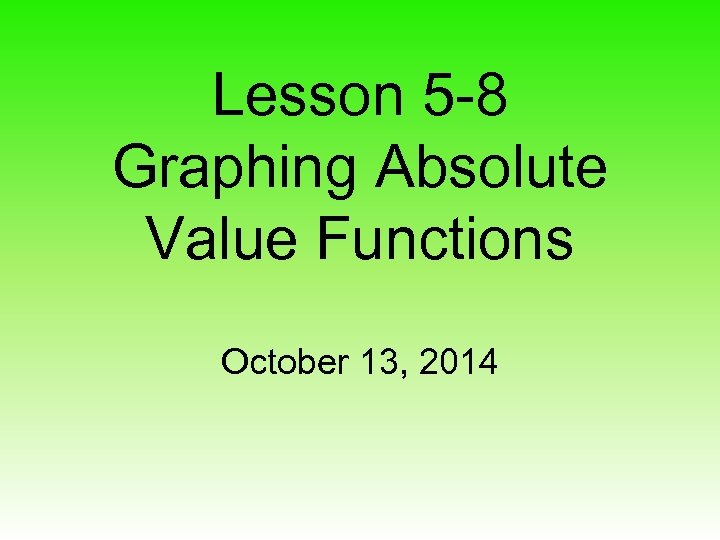Lesson 5 -8 Graphing Absolute Value Functions October 13, 2014Daily Learning Target • I will graph and write an equation of an absolute value function • I will describe the translation of the graph of an absolute value function.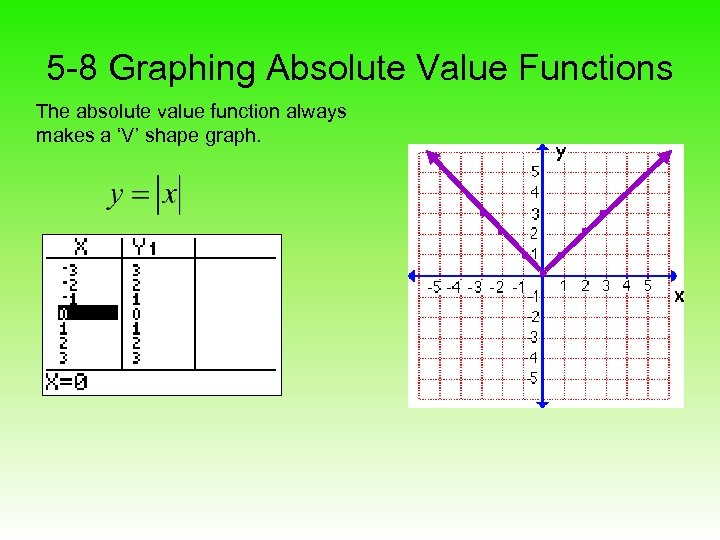5 -8 Graphing Absolute Value Functions The absolute value function always makes a ‘V’ shape graph.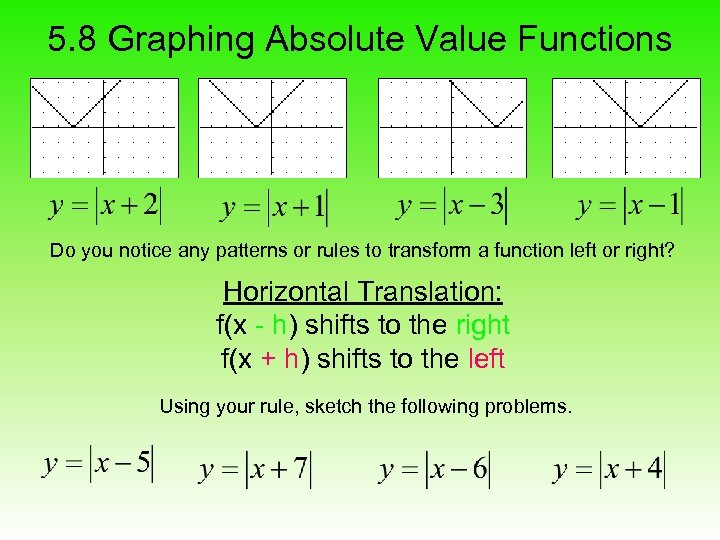5. 8 Graphing Absolute Value Functions Do you notice any patterns or rules to transform a function left or right? Horizontal Translation: f(x - h) shifts to the right f(x + h) shifts to the left Using your rule, sketch the following problems.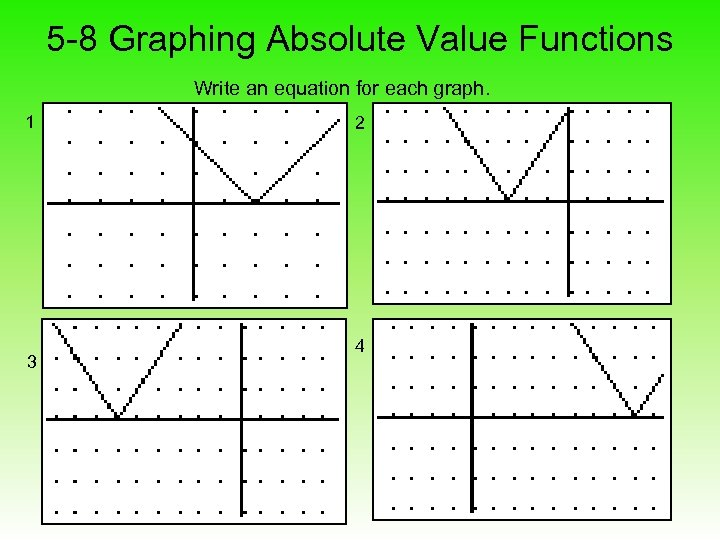5 -8 Graphing Absolute Value Functions Write an equation for each graph. 1 3 2 4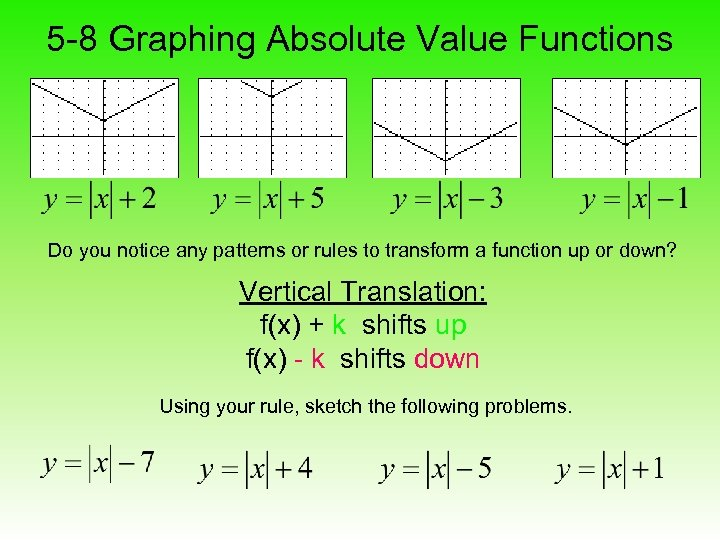5 -8 Graphing Absolute Value Functions Do you notice any patterns or rules to transform a function up or down? Vertical Translation: f(x) + k shifts up f(x) - k shifts down Using your rule, sketch the following problems.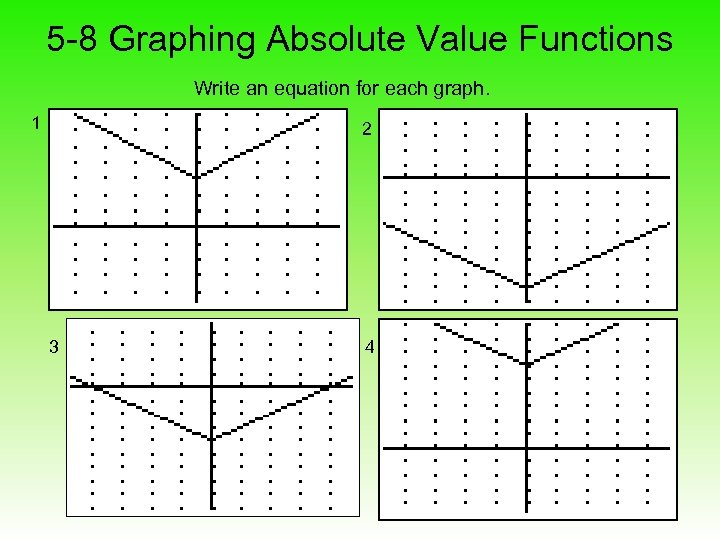5 -8 Graphing Absolute Value Functions Write an equation for each graph. 1 2 3 4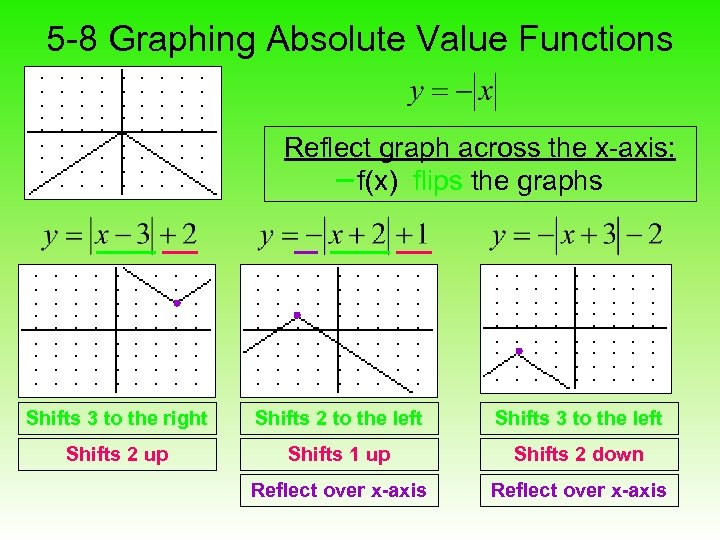5 -8 Graphing Absolute Value Functions Reflect graph across the x-axis: f(x) flips the graphs Shifts 3 to the right Shifts 2 to the left Shifts 3 to the left Shifts 2 up Shifts 1 up Shifts 2 down Reflect over x-axis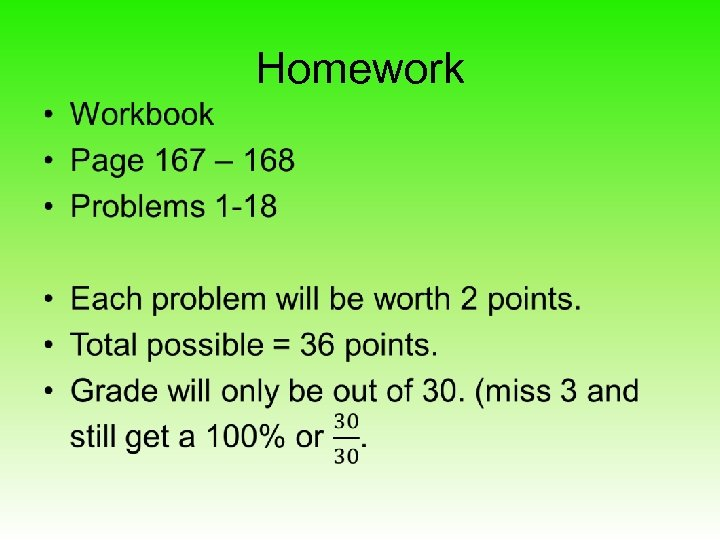Homework •